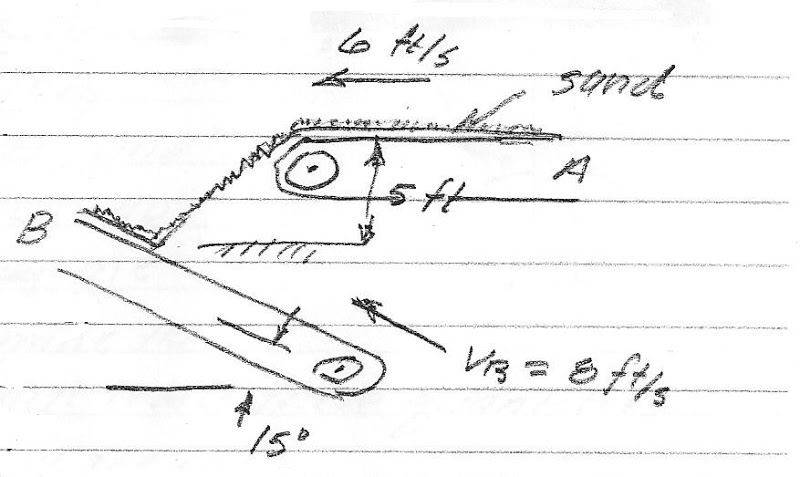# Relative motion on a conveyor belt

Gold Member

## Homework Statement

Belt A conveys sand with a velocity of 6.0 ft/s (constant). The velocity of belt B is 8 ft/s (constant). Determine the velocity of the sand relative to B as it lands on belt B. Answer: VS/B=20.1 ft/s at 85.1 degrees## Homework Equations

VS/B = VS-VB
(Velocity of sand relative to belt B = velocity of sand as it hits belt B - velocity of belt B)

## The Attempt at a Solution

VB=8cos(15°)+8sin(15°) = -7.727$$\hat{i}$$+2.071$$\hat{j}$$(ft/s)

My trouble is with finding VS. I know that on the horizontal section of belt A, the sand's velocity (VS) is -6$$\hat{i}$$+0$$\hat{j}$$(ft/s).

When the sand hits the bend where belt A is not horizontal anymore, but starts to bend into belt B, the velocity of the sand changes.

I think I'm stuck on the trig of what the angle is and how the 5ft contributes to finding the correct angle that the sand runs into belt B.